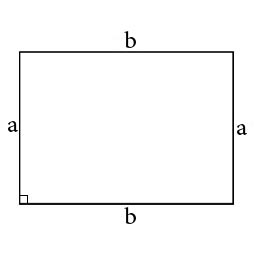# Perimeter of a rectangle calculator.

You could easily calculate rectangle perimeter by our online calculator.Length of leg a: Length of leg b:

If you want to calculate rectangle's perimeter it is needed to know the length of two it's sides which are placed orthogonal. Keep in mind that opposite sides are equal. Next just multiply a side's length by 2 and b side's length by 2 and, next sum derived values up. This way we can make formula for rectangle's perimeter:

P = 2a+2b.

### The perimeter of a rectangleHOME
RESOURCES